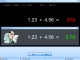RekenTest 4.4.1 Practice arithmetic skills, for kids and professionals Freeware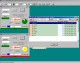CalculPro 1.2.1 CalculPro to practise mental arithmetic’s or fractions. Shareware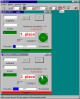Calcul 5.2.WIN32 CALCUL to practise mental arithmetic’s or fractions. Freeware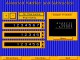Animated Arithmetic 1.0 Teaches addition, subtraction, multiplication and division for 1st - 4th grades. Shareware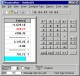NumberMate 2.1.3 Arithmetic and scientific calculator records calculations on tapes you can edit Shareware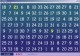Math Worm 2.0 A math puzzle where your worm navigates a maze completing arithmetic equations. Shareware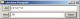Calculator Prompter 2.7 Calculator Prompter is a math expression calculator. It is a handy and useful. Freewareapfloat Lib 2.41 Apfloat is a C++ arbitrary precision arithmetic package. Freeware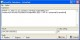ScienCalc 1.3.22 ScienCalc is a convenient and powerful scientific calculator. SharewareProportions 0.1.1 Proportions is a small widget to easily calculate arithmetic proportions. Freeware